College Physics : Ohm's Law and Current

Example Questions

Example Question #21 : Electromagnetics

What size resistor should be connected across the terminals of a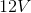battery to produce a current of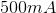?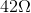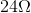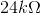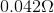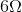Explanation:

Ohm's law is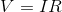.

In this case,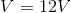and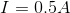.

Solving for the resistance,, we get: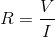Substitute known values and solve for the unknown resistance: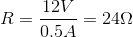Example Question #105 : College Physics

A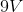battery is connected to a small heater with constant resistance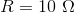. How much current flows through the heater?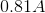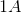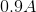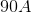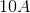Explanation:

Ohm's law relates voltage to current and resistance,. To find the current, we simply divide the voltage by the resistance and get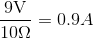Example Question #106 : College Physics

Suppose that a circuit with a single resistor is connected to a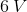battery. If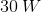of power is generated across this resistor, what is its resistance?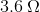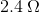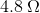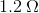Explanation:

For this problem, let's first take note of what we know and what we don't. We're given voltage and power, but we're not given resistance or current. Since we have two unknowns, we're going to need two equations.

One of the equations that we can use is the power generated by current flowing through a resistor.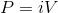In addition, we also need to use Ohm's law.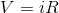With these two equations in mind, we can relate them to one another by isolating the variable for current in one equation and then substituting it into the other one.

Manipulating Ohm's law: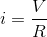Then substituting this into the power equation: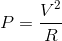And rearranging to isolate the variable for resistance: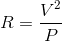Now we just need to plug in the values from the question stem to obtain the answer: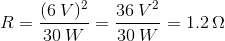Example Question #1 : Ohm's Law And Current

The picture below shows the electron energy levels of a hydrogen atom.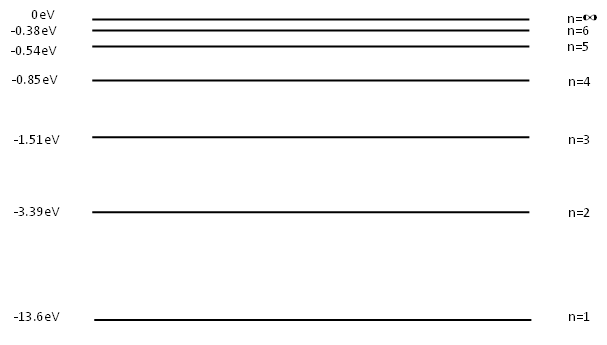If an electron is excited to the 5th energy level, how many different transitions can it make to return to ground state?

Zero

One

Two

Three

Ten

Ten

Explanation:

Electrons don't always drop from the top to the bottom, sometimes they can make 2 or more transitions during the journey. For example, it could drop to the n=4 then to the n=1 levels. Or n=4 to n=2 then n=1. So when you count up all the possible transitions that can be made between n=5 and n=1, you get 10.

Example Question #108 : College Physics

Use this circuit diagram for the next question.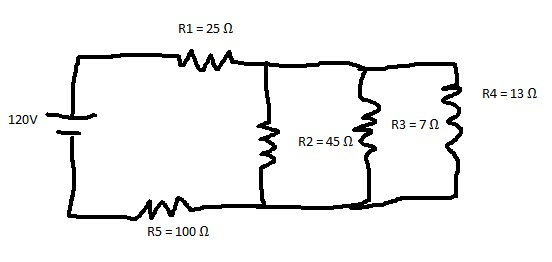What is the current traveling through the R2 resistor?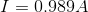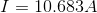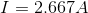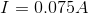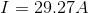Explanation:

So this problem has several steps to it. We need to think backwards. Ultimately we need to know the current going through R2. We will be using Ohms law so we need to know the voltage going through just that resistor.

Because it is in parallel with R3 and R4, all three must have the same voltage. However since it is in series with 2 other resistors, it will not be the voltage from the battery.

Lets reduce the parallel circuit to 1 resistor. Using the formula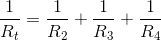, then remembering to invert the answer yields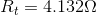.

That resistance is in series with R1 and R5 so to find the current going through each of them we need to add the resistances and use Ohm's law again.

Doing so yields a current of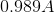. This is the current going through the circuit. Now we can find the voltage drop of just our equivalent parallel resistor.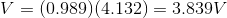.

So all three of the resistors that are in parallel have a voltage of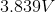going through them.

Now we can go all the way back to the R3 resistor knowing that it has a resistance of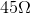and a voltage of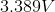. Use Ohm's Law one more time to solve for the current of the R3 resistor.

All College Physics Resources